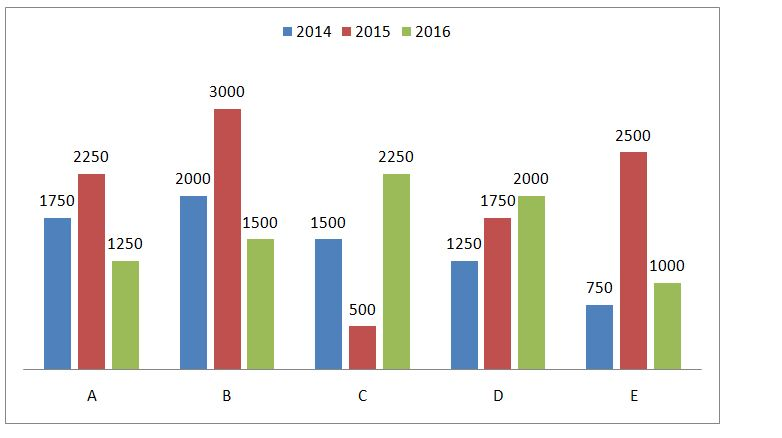# IBPS Clerk Prelims Quantitative Aptitude Questions 2019 (Day-21)

Dear Aspirants, Our IBPS Guide team is providing new series of Quantitative Aptitude Questions for IBPS Clerk Prelims 2019 so the aspirants can practice it on a daily basis. These questions are framed by our skilled experts after understanding your needs thoroughly. Aspirants can practice these new series questions daily to familiarize with the exact exam pattern and make your preparation effective.

Ensure Your Ability Before of Exam – Take IBPS Clerk Prelims Free Mock test

[WpProQuiz 7459]

Data Interpretation

Directions (Q. 1 – 5): Study the following information carefully and answer the given questions?

The following bar graph shows the total number of items produced by five different companies in three different years.1) Number of items produced by company A, C and E together in 2014 is approximately what percentage of the number of items produced by company B, C and D together in 2015?

a) 70 %

b) 80 %

c) 55 %

d) 90 %

e) 65 %

2) Find the average number of items produced by company A and B together in all the given years together?

a) 5875

b) 5500

c) 5250

d) 5625

e) None of these

3) Number of items produced by all the given companies in 2014 is approximately what percentage more than the number of items produced by company C in all the years together?

a) 40 %

b) 80 %

c) 70 %

d) 50 %

e) 60 %

4) Find the difference between the total number of items produced by all the companies together in 2015 to that in 2016?

a) 2000

b) 5000

c) 3000

d) 4000

e) None of these

5) Find the respective ratio between the total number of items produced by the company B and E in 2014 to that of the total number of items produced by A and D in 2016?

a) 12 : 19

b) 13 : 11

c) 19 : 12

d) 11 : 13

e) None of these

Simplification

Directions (Q. 6 – 10): what value should come in place of question mark (?) in the following questions?

6) 35 % of 620 + (3/11) of 20350 – 17 % of 800 = ?

a) 4815

b) 5631

c) 4652

d) 5859

e) None of these

7) ? % of 600 = 2562 + (672 – 342) × 42 ÷ 11

a) 3136

b) 3574

c) 2352

d) 2548

e) None of these

8) (5274 + 1442) ÷ 23 + 492 – 52 = ?

a) 3178

b) 2153

c) 2641

d) 3569

e) None of these

9) 6 3/8 + 5 ¾ – 4 ½ – 3 1/4 = ? – 7 ½

a) 13 3/4

b) 12 6/11

c) 11 7/8

d) 9 ½

e) None of these

10) (3/7) of 3164 – 135 × 12 ÷ 5 = ?

a) 1032

b) 1156

c) 1278

d) 1324

e) None of these

Directions (1-5):

Number of items produced by company A, C and E together in 2014

= > 1750 + 1500 + 750 = 4000

Number of items produced by company B, C and D together in 2015

= > 3000 + 500 + 1750 = 5250

Required percentage = (4000 / 5250) * 100 = 76.19 % = 80 %

The total number of items produced by company A in all the given years together

= > 1750 + 2250 + 1250 = 5250

The total number of items produced by company B in all the given years together

= > 2000 + 3000 + 1500 = 6500

Required average = (5250 + 6500) / 2 = 11750 / 2 = 5875

Number of items produced by all the companies in 2014

= > 1750 + 2000 + 1500 + 1250 + 750 = 7250

Number of items produced by company C in all the years together

= > 1500 + 500 + 2250 = 4250

Required percentage = [(7250 – 4250) / 4250] * 100 = 70.58% = 70 %

Total number of items produced by all the companies in 2015

= > 2250 + 3000 + 500 + 1750 + 2500 = 10000

Total number of items produced by all the companies in 2016

= > 1250 + 1500 + 2250 + 2000 + 1000 = 8000

Required difference = 10000 – 8000 = 2000

Total number of items produced by companies B, E in 2014

= > 2000 + 750 = 2750

Total number of items produced by companies A and D in 2016

= > 1250 + 2000 = 3250

Required ratio = 2750 : 3250 = 11 : 13

Directions (6-10):

(35/100) * 620 + (3/11) * 20350 – (17/100) * 800 = x

x = 217 + 5550 – 136

x = 5631

(x/100) * 600 = 2562 + [(67 + 34) (67 – 34)] * (42/11)

6x = 2562 + (101 * 33) * (42 / 11)

6x = 2562 + 12726

6x = 15288

x = 2548

6716/23 + 492 – 52 = x

x = 292 + 2401 – 52

x = 2641

6 3/8 + 5 ¾ – 4 ½ – 3 ¼ + 7 ½ = x

x = (6 + 5 – 4 – 3 + 7) (3/8 + ¾ – ½ – 1/4 + ½)

x = 11 [(3 + 6 – 2)/8

x = 11 (7/8)